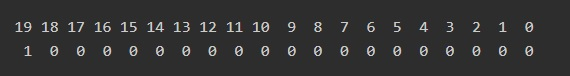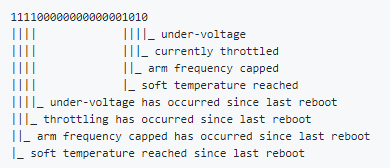# 5.9: Monitoring your Raspberry Pi

$$\newcommand{\vecs}{\overset { \rightharpoonup} {\mathbf{#1}} }$$ $$\newcommand{\vecd}{\overset{-\!-\!\rightharpoonup}{\vphantom{a}\smash {#1}}}$$$$\newcommand{\id}{\mathrm{id}}$$ $$\newcommand{\Span}{\mathrm{span}}$$ $$\newcommand{\kernel}{\mathrm{null}\,}$$ $$\newcommand{\range}{\mathrm{range}\,}$$ $$\newcommand{\RealPart}{\mathrm{Re}}$$ $$\newcommand{\ImaginaryPart}{\mathrm{Im}}$$ $$\newcommand{\Argument}{\mathrm{Arg}}$$ $$\newcommand{\norm}{\| #1 \|}$$ $$\newcommand{\inner}{\langle #1, #2 \rangle}$$ $$\newcommand{\Span}{\mathrm{span}}$$ $$\newcommand{\id}{\mathrm{id}}$$ $$\newcommand{\Span}{\mathrm{span}}$$ $$\newcommand{\kernel}{\mathrm{null}\,}$$ $$\newcommand{\range}{\mathrm{range}\,}$$ $$\newcommand{\RealPart}{\mathrm{Re}}$$ $$\newcommand{\ImaginaryPart}{\mathrm{Im}}$$ $$\newcommand{\Argument}{\mathrm{Arg}}$$ $$\newcommand{\norm}{\| #1 \|}$$ $$\newcommand{\inner}{\langle #1, #2 \rangle}$$ $$\newcommand{\Span}{\mathrm{span}}$$$$\newcommand{\AA}{\unicode[.8,0]{x212B}}$$

## Monitoring Clock Frequency, Voltage and Temperature

Using the vcgencmd command we can get some useful information about our Raspberry Pis.

Open Terminal and type the following commands:

vcgencmd measure_clock core
vcgencmd measure_volts core
vcgencmd measure_temp

The output should look close to this one:Figure $$\PageIndex{1}$$: Sample output

According to Raspberry Pi Documentation, the official operating CPU temperature limit is 85°C. When the core temperature reaches 80°C you will see a warning icon with a half-filled thermometer.Figure $$\PageIndex{2}$$: This icon indicates temperatures between 80°C and 85°C

At this point various frequencies and sometimes voltages used on the chip (ARM*, GPU**) are reduced. It is necessary to reduce the amount of heat generated and keep the CPU temperature down.

If the CPU temperature reaches 85°C the ARM cores and the GPU will be throttled back. You will see another warning icon - a fully filled thermometer.Figure $$\PageIndex{3}$$: This icon indicates temperatures of 85°C and higher

## Using get_throttled Command

Now let’s look at another parameter we need. In your terminal type the following command and hit enter

vcgencmd get_throttled

You should see something like this (the numbers you get might be different)Figure $$\PageIndex{4}$$: get_throttled command and output

But what does this mean? Let's check Raspberry Pi Documentation where we can find this information.

Table $$\PageIndex{1}$$: Bit Pattern
Bit Meaning
0 Under-voltage detected
1 Arm frequency capped
2 Currently throttled
3 Soft temperature limit active
16 Under-voltage has occurred
17 Arm frequency capping has occurred
18 Throttling has occurred
19 Soft temperature limit has occurred

It helped some, but it is still not clear what those numbers mean and how to use this table. We need to look deeper. We are going to use Python to convert this value to an integer (it is a string by default) and then to a binary number.

1. Open Thonny

2. Convert the string you have to an integer

a=int('0x80000', 16) # base set to 16 for hexadecimal
print ('a =',a)

In this code 16 is a base we need to use to let Python know that it is a hexadecimal. You can also set the base to 0 and let Python figure it out based on the 0x in the number.

b=int('0x80000', 0) # base set to 0 for Python to figure it out
print ('b =',b)

Both numbers will be the same, so you can choose which way is more convenient for you. We will use the first example.

3. Now it’s time to convert the number we have to a binary number. Here is the full code:

a=int('0x80000', 16)
print ('a =',a)
binary = bin(a)
print(a, '=', binary)

4. The output will differ depending on the original value, but here is the output for our example:Figure $$\PageIndex{5}$$: Binary number

To interpret this value we need to number the bits. Remember that numbering starts with zero and goes right to left (ignore 0b):Figure $$\PageIndex{6}$$: Number the bits

It is clear now that bit 19 is set (1 means on, 0 means off), which according to Table 1 indicates that Soft Temperature Limit has occurred at some point. The bits chart below was found in one of the Raspberry Pi firmware commit comment on GitHub. It illustrates the meaning of each bit and might be easier to use.Figure $$\PageIndex{7}$$: Bits Chart

Soft Temperature Limit was introduced for the Raspberry Pi 3B+ Model. When the temperature reaches 60°C (default Soft Temperature Limit) the clock speed goes from 1.4GHz to 1.2GHz and the operating voltage is also reduced slightly. It is necessary to reduce the rate at which the temperature is increasing. Basically, you are trading a shorter period at 1.4GHz for a longer period at 1.2GHz. This gives you more time before the Raspberry Pi reaches the hard limit of 85°C.

______